# Some Particular Types of Quadrilaterals

We have heard and read about squares and rectangles, which are known as polygons with 4 sides and 4 vertices.

They are also known as quadrilaterals.

However, do you know that there are other types of quadrilaterals too?

Once upon a time, there were 4 children who were flying kites in a garden.

They looked at the shapes of their kites very carefully and found out that all the four kites have 4 sides and 4 vertices.Let's learn about some particular types of quadrilaterals in this lesson by learning about their names and properties with the help of some solved examples and a few interactive questions for you to test your understanding.

Let's begin!

## Lesson Plan

 1 What Are the Particular Types of Quadrilaterals? 2 Important Notes 3 Thinking Out of the Box! 4 Solved Examples 5 Interactive Questions

The word "quadrilateral" is derived from two Latin words, "Quadri" which means "four," and "Latus" which means "side."

Hence, a quadrilateral is a polygon with 4 sides.

A quadrilateral can be defined in two ways:

• A quadrilateral is a closed shape that is obtained by joining four points among which any three points are non-collinear.
• A quadrilateral is a closed 2D shape with four vertices and four sides.

Observe the two examples shown below.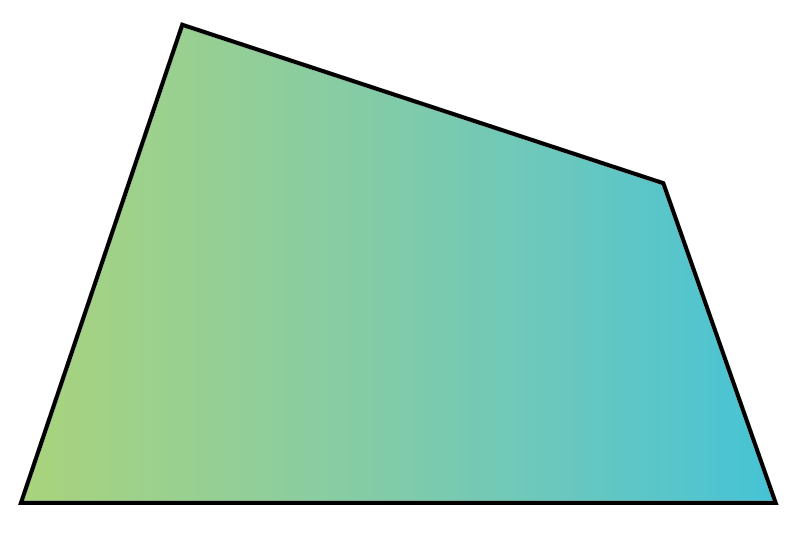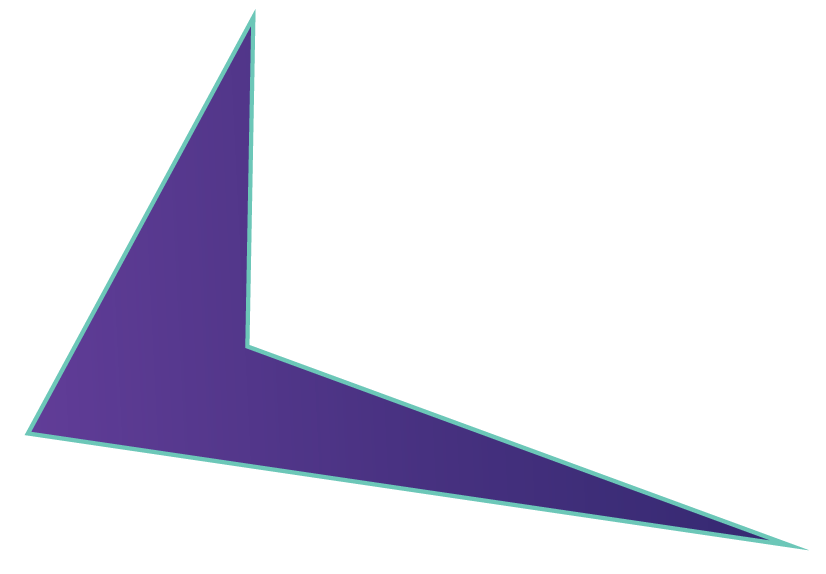## What Are the Particular Types of Quadrilaterals?

There are majorly six types of quadrilaterals.

Observe the relationship between each type of quadrilateral from the given chart.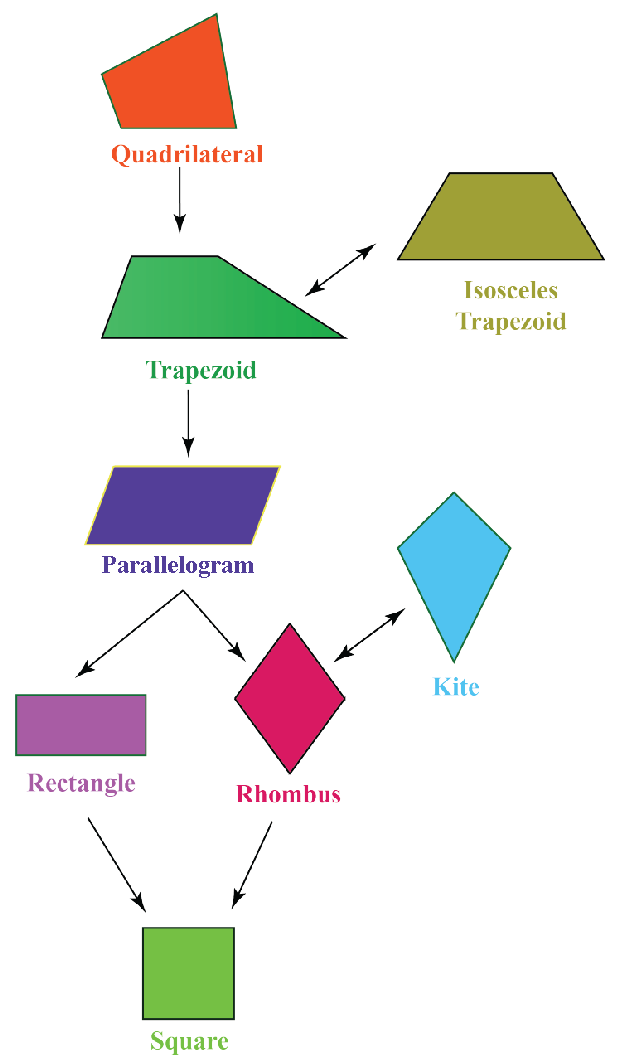Observe the table given below to have a basic idea of the shapes of different types of quadrilaterals.

 S.No. Quadrilateral Shape 1 Square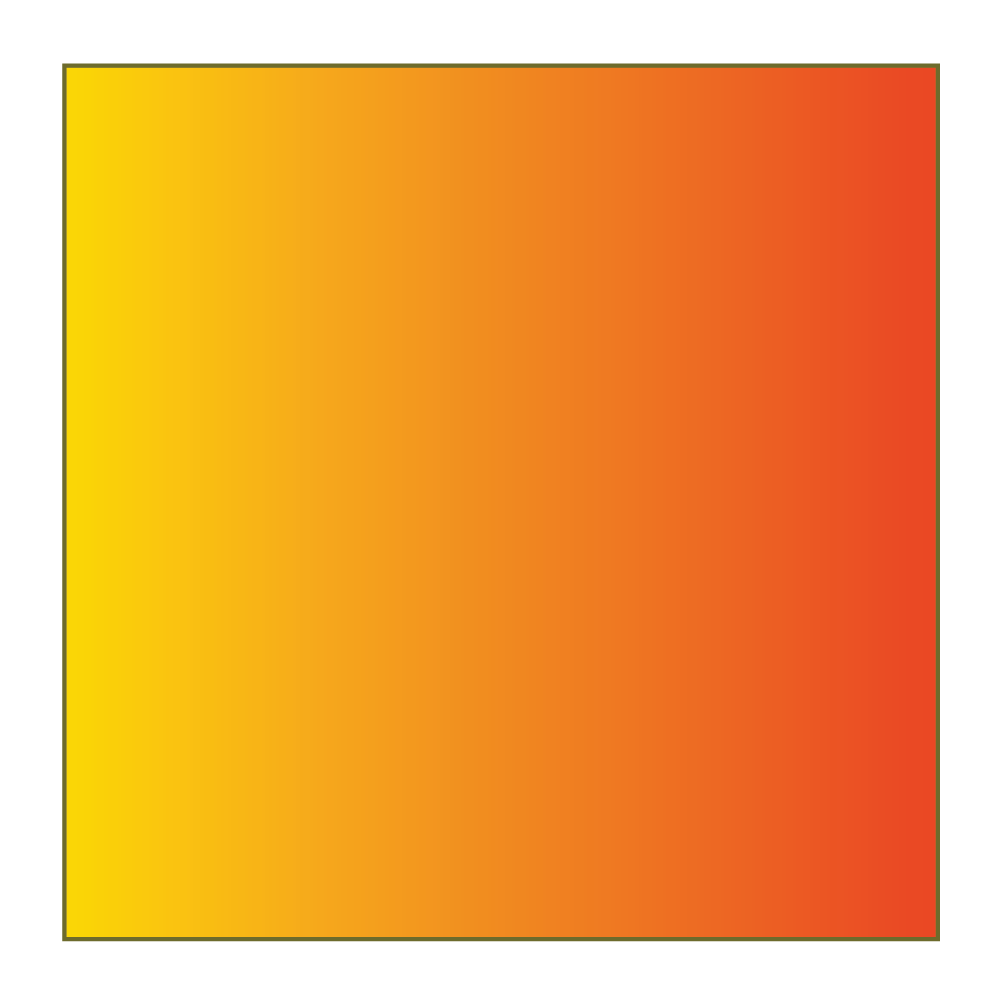2 Rectangle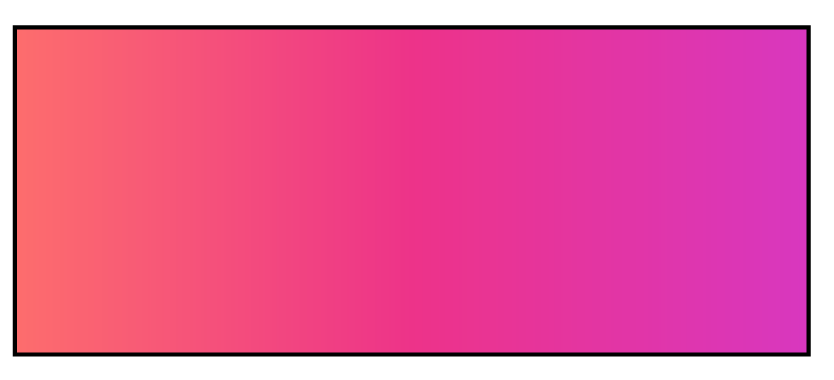3 Parallelogram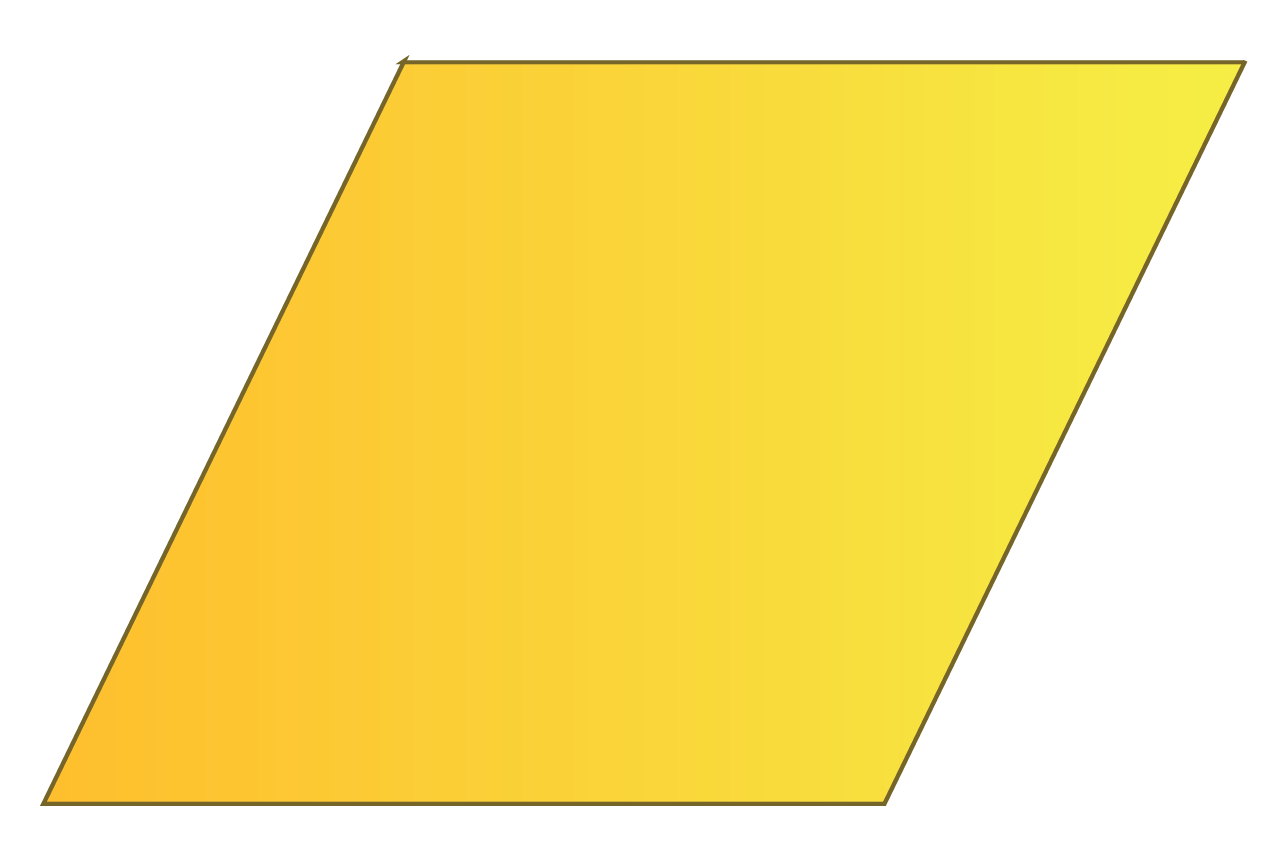4 Trapezoid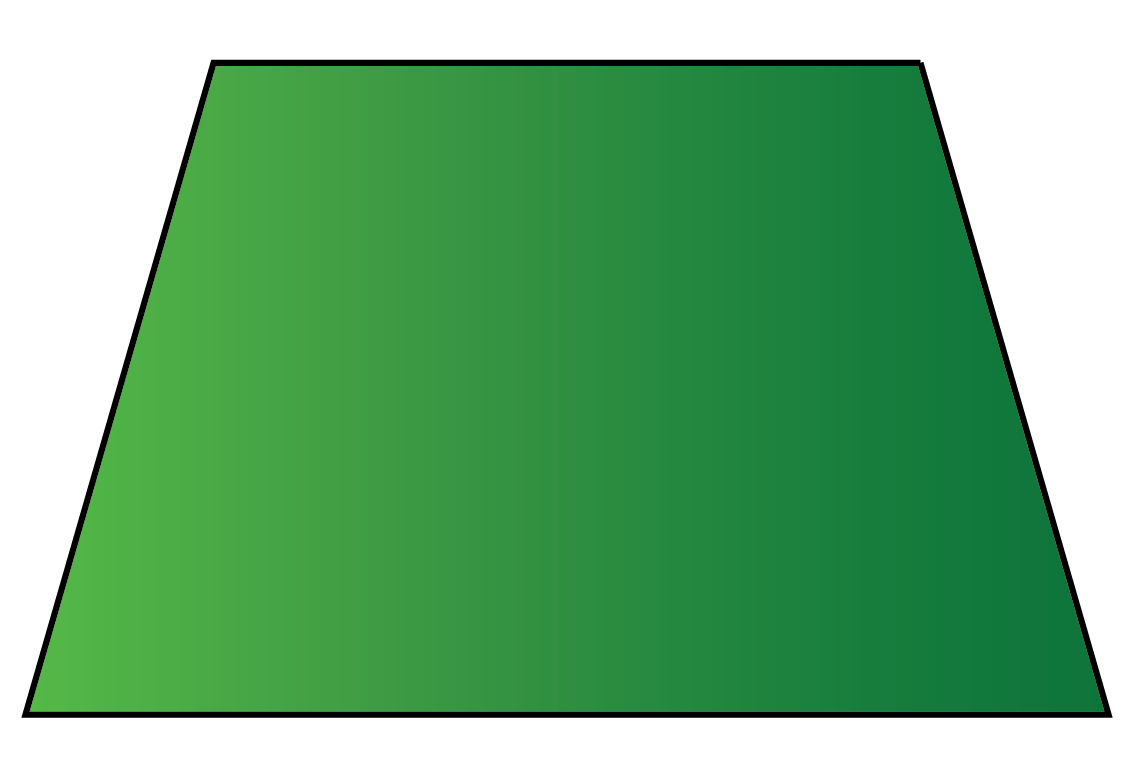5 Rhombus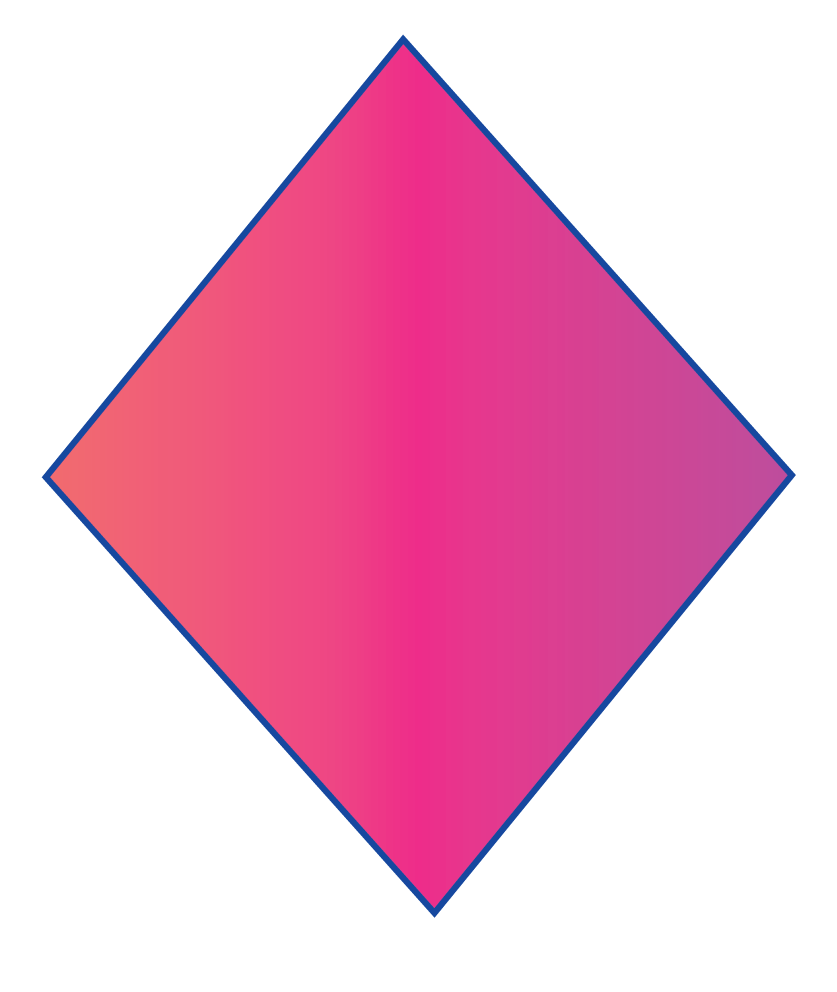6 Kite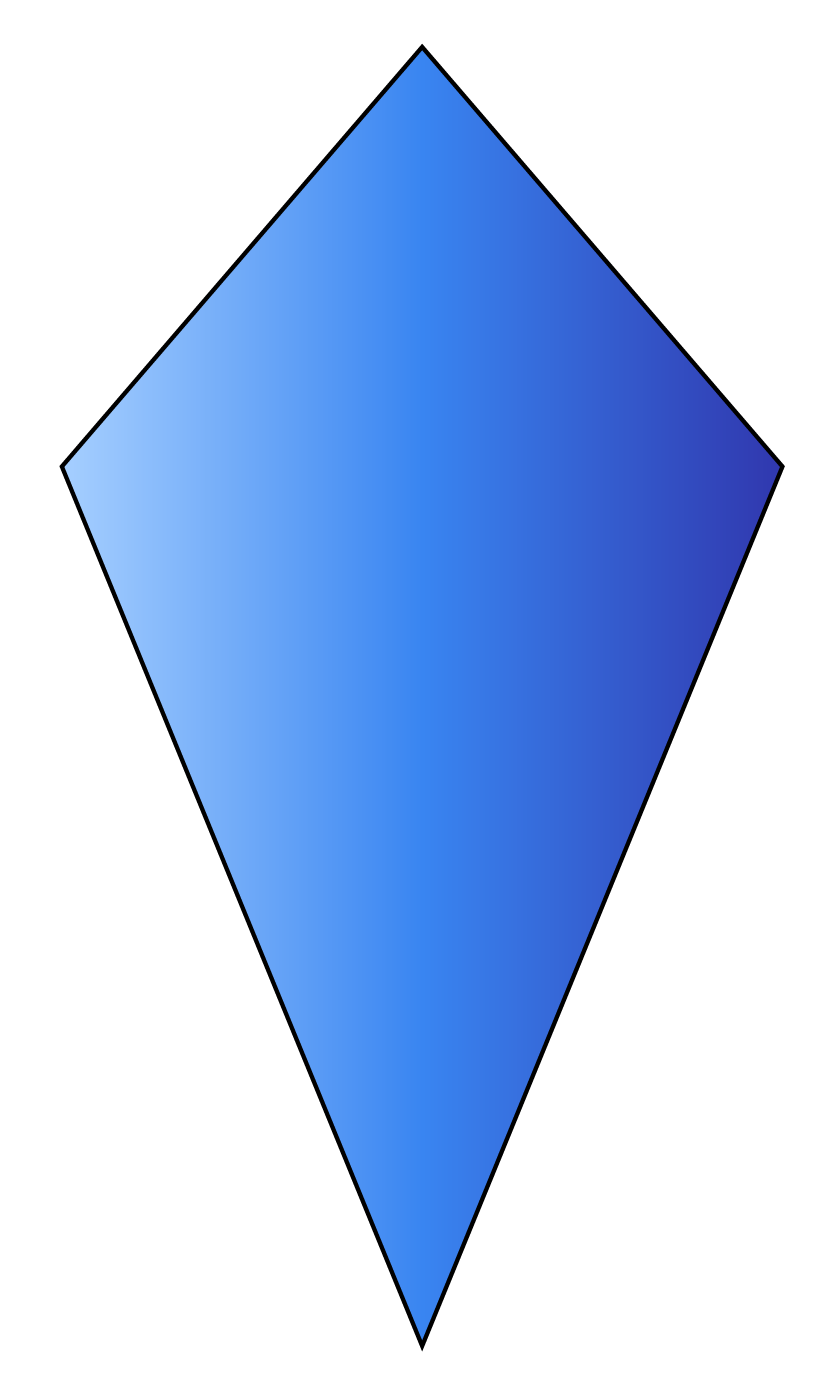### 1. Is rhombus a kite?

Yes, a rhombus is a kite because the adjacent sides in a rhombus are equal.

Quadrilaterals are 2D closed shapes having 4 sides and 4 vertices.

### 3. What are the 8 types of quadrilaterals?

The eight types of quadrilaterals are square, rectangle, parallelogram, rhombus, trapezoid, isosceles trapezoid, kite, and irregular quadrilaterals.

### 4. Can we say that diamond shape is a quadrilateral?

Yes, we usually represent the shape of a diamond with a rhombus or a kite. So, a diamond shape is a quadrilateral.

### 5. Is every square a rectangle?

Yes, every square is a rectangle as it satisfies all the properties of a rectangle.

### 6. Is every rhombus a parallelogram?

Yes, every rhombus is a parallelogram because its opposite sides are parallel and equal; the opposite angles are equal and the diagonals bisect each other.

### 7. Can a parallelogram be considered as a rectangle?

A parallelogram can be considered as a rectangle only when all four interior angles in a parallelogram are of 90° each.

More Important Topics
Numbers
Algebra
Geometry
Measurement
Money
Data
Trigonometry
Calculus